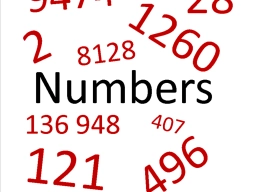# Solve 17

Solve a reciprocal equation:

18 36/64=9:n

n =  16/33

### Step-by-step explanation:Did you find an error or inaccuracy? Feel free to write us. Thank you!

Showing 1 comment:
math Peter
that is useful I guess

Tips for related online calculators
Need help with mixed numbers? Try our mixed-number calculator.
Do you have a linear equation or system of equations and looking for its solution? Or do you have a quadratic equation?